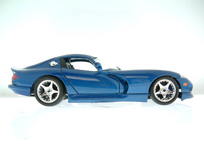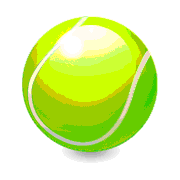Forces

Q1-3. Which of these forces....

1. ...does a fridge magnet use?

2. ...keeps the Moon in orbit around the Earth?

3. ...stops things moving?

4.This car has been shaped so that it has less friction than a school bus.We say it has been ...

• A. smoothed
• B. de-blocked
• C. frictionised
• D. streamlined

5. Here are 4 things you can wear on your feet. For which one do we want the least friction?

• B. skis
• C. running shoes
• D. hiking boots

6. Forces can do many things to a moving tennis ball. Which one of these can it not do?

• A. Change its mass
• B. Change its shape
• C. Change its speed
• D. Change its directionHere is a picture of a car that is moving - it has 4 forces acting on it. Force Q is equal in size to S, and force P is equal to R.7. What will the car do?

• A. Speed up
• B. Stay at the same speed
• C. Slow down
• D. Stop

8. What is the name given to forces like this that cancel out?

• A. Balanced forces
• B. Equalised forces
• C. Zeroed forces
• D. Net forces
 9. To make the car speed up , force ? P Q R S must increase . 10. Air resistance is shown by force ? P Q R S .